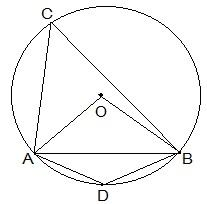# A chord of a circle is equal to the radius of the circle. Find the angle subtended by the chord at a point on the minor arc and also at a point on the major arc.The chord AB is equal to the radius of the circle.

OA and OB are the two radii of the circle.

From ΔOAB.

AB = OA = OB = radius of the circle.

ΔOAB is an equilateral triangle.

Therefore,

AOC = 60°

And, ACB = ½ AOB

So, ACB = ½ × 60° = 30°

Now, ACBD is a cyclic quadrilateral,

ADB +ACB = 180° (Since they are the opposite angles of a cyclic quadrilateral)

So, ADB = 180°-30° = 150°

Hence, the angle subtended by the chord at a point on the minor arc and also at a point on the major arc is 150° and 30° respectively.(0)(0)